# 10-1100/Homework Assignment 3

Jump to: navigation, search

This assignment is due at class time on Tuesday, November 16, 2010.

### Solve the following questions

Problem 1. (Selick) Show that any group of order 56 has a normal Sylow-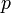$p$ subgroup, for some prime$p$ dividing 56.

Problem 2. (Qualifying exam, May 1997) Let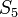$S_5$ act on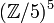$({\mathbb Z/5})^5$ by permuting the factors, and let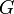$G$ be the semi-direct product of$S_5$ and$({\mathbb Z/5})^5$.

1. What is the order of$G$?
2. How many Sylow-5 subgroups does$G$ have? Write down one of them.

Problem 3. (Selick) Show that the group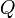$Q$ of unit quaternions (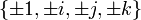$\{\pm 1, \pm i, \pm j, \pm k\}$, subject to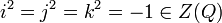$i^2=j^2=k^2=-1\in Z(Q)$ and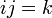$ij=k$) is not a semi-direct product of two of its proper subgroups.

Problem 4. (Qualifying exam, September 2008) Let$G$ be a finite group and$p$ be a prime. Show that if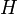$H$ is a$p$-subgroup of$G$, then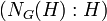$(N_G(H):H)$ is congruent to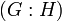$(G:H)$ mod$p$. You may wish to study the action of$H$ on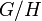$G/H$ by multiplication on the left.

Problem 5. (easy)

1. Prove that in any ring,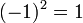$(-1)^2=1$.
2. Prove that even in a ring without a unit,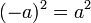$(-a)^2=a^2$.

(Feel free to do the second part first and then to substitute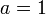$a=1$).

Problem 6.

1. (Qualifying exam, April 2009) Prove that a finite integral domain is a field.
2. (Qualifying exam, September 2008) Prove that in a finite commutative ring, every prime ideal is maximal.

Problem 7. (Dummit and Foote) A ring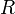$R$ is called a Boolean ring if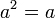$a^2=a$ for all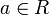$a\in R$.

1. Prove that every Boolean ring is commutative.
2. Prove that the only Boolean ring that is also an integral domain is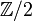${\mathbb Z}/2$.